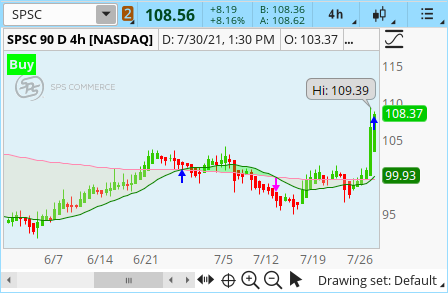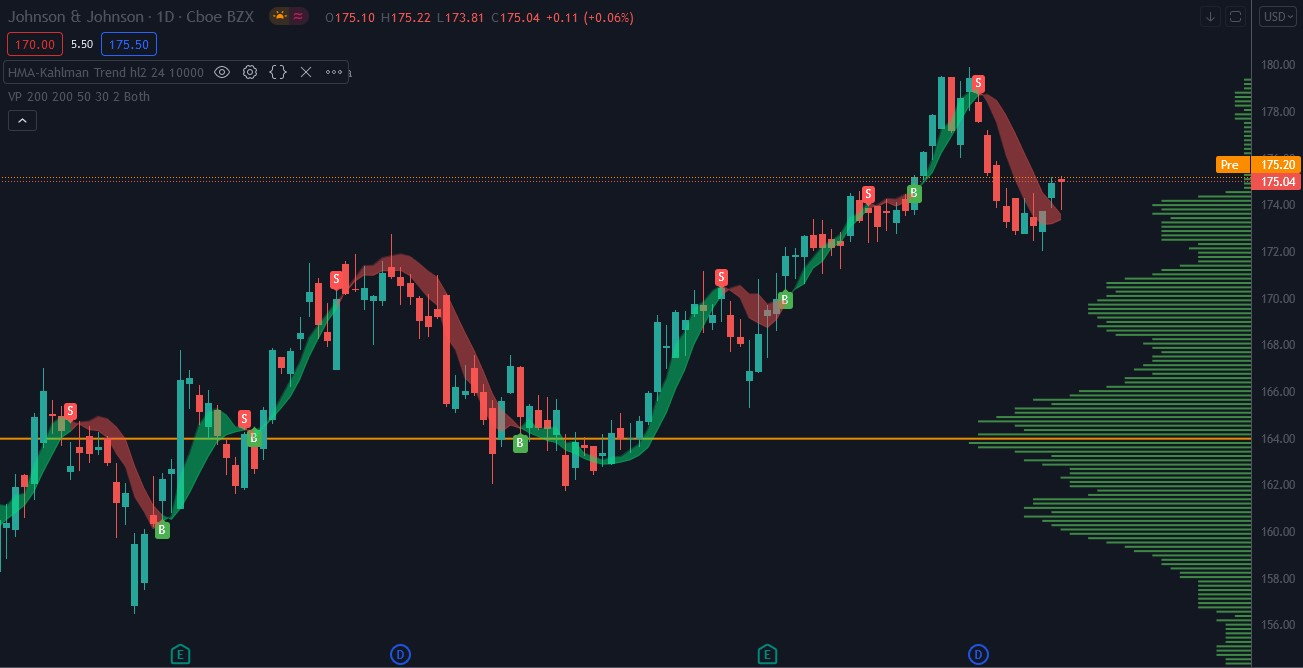# Kalman Filter

##### New member
Anyone try coding the Kalman Filter? EasyLanguage version is out there but I have yet to see someone able to convert it to ThinkScript.

Solution
@bp805
Code:
``````input src = hl2;
input length = 24;
input showcross = yes;
input gain = 10000;
input k = yes;

script hma {
input _src = 0;
input _length = 0;
def avgType = AverageType.WEIGHTED;

plot return = MovingAverage(avgType, (2 * MovingAverage(avgType, _src, _length /
2)) - MovingAverage(avgType, _src, _length), Round(Sqrt(_length)));
}

script hma3 {
input _length = 0;
def p = _length / 2;
def avgType = AverageType.WEIGHTED;

plot return = MovingAverage(avgType, 3 * MovingAverage(avgType, close, p / 3) -
MovingAverage(avgType, close, p / 2) - MovingAverage(avgType,
close, p), p);
}

script kahlman {
input x = 0;
input g = 0;
def...``````
Here's a Kalman that I have from a while back

input n = 21;

plot Kalman = WMA(((WMA(close, 3) * 3) + (WMA(close, 2) * 2) + close + Average(close, n)) / 7, n);

# Simple Kalman Filter Compared to different Averages
# Mobius
# 2.22.2016

input n = 8;
input Kalman = yes;
input SMA = yes;
input EMA = yes;
input Weighted_MA = yes;

plot Data = WMA(((close * 3) + (close * 2) + close + Average(close, n)) / 7, n);
Data.SetDefaultColor(Color.Cyan);
Data.SetHiding(!Kalman);

plot avg = Average(close, n);
avg.SetDefaultColor(Color.Yellow);
avg.SetHiding(!SMA);

plot ExAvg = ExpAverage(close, n);
ExAvg.SetDefaultColor(Color.Red);
ExAvg.SetHiding(!EMA);

plot WtAvg = WMA(close, n);
WtAvg.SetDefaultColor(Color.Green);
WtAvg.SetHiding(!Weighted_MA);

Don't know why, but I have Kolmogorov-Feller linked to Kalman - curious cross-overs

declare lower;
input n = 20;
def s = fold i = 1 to n with d = 0 do d+log(i);
def f = fold j = 1 to n with m = 0 do m+log(getvalue(close,j,n))*log(j);
def k = fold g = 1 to n with c = 0 do c+power(log(g),2);
def alpha = ((Sum(log(Close),n)*s) - (n*f))/((s*s)-n*k);
def a = exp(((Sum(Close,n)-alpha*s)/n)/1000);
plot al = a;

al.assignvaluecolor(if al > al then color.blue else if al < al then color.red else color.white);
plot trig = al;

#Blackledge "FINANCIAL FORECASTING USING THE
#KOLMOGOROV-FELLER EQUATION"
#growex

plot Data = WMA(((a * 3) + (a * 2) + a + Average(a, n)) / 7, n);
Data.SetDefaultColor(Color.Cyan);

Hi all, trying to create a cross and having challenges.

original code:

input n = 21;

plot Kalman = WMA(((WMA(close, 3) * 3) + (WMA(close, 2) * 2) + close + Average(close, n)) / 7, n);

Trying to do a cross above from kalman to SMA with an arrow alert and sound when it crosses. Below is how far i got and keeps giving me error. Can someone helpKalman(100) crosses above SimpleMovingAvg("length" = 200)."SMA"

thank you

@tradebook Could you explain how you are using this indicator?
Kalman SMA200 Cross with Arrows, Label, and Alerts
Ruby:
``````# ########################################################
# Kalman SMA200 cross

input n = 21;
input showLabel = yes ;
DefineGlobalColor("LitePink", CreateColor (225, 220, 180)) ;

plot Kalman = WMA(((WMA(close, 3) * 3) + (WMA(close, 2) * 2) + close + Average(close, n)) / 7, n);
plot avg200 = MovingAverage(AverageType.SIMPLE, close, 200);

plot UpArrow = if  Kalman crosses above avg200  then low else double.NaN ;
UpArrow.SetPaintingStrategy(PaintingStrategy.ARROW_up);
UpArrow.SetDefaultColor(color.blue) ;

plot DownArrow = if  Kalman crosses below avg200 then high else double.NaN ;
DownArrow.SetPaintingStrategy(PaintingStrategy.ARROW_down);
DownArrow.SetDefaultColor(color.magenta) ;

if Kalman crosses above avg200 then "Buy"  else
if Kalman crosses below avg200 then "Sell" else "Kalman: " +round(Kalman,1),
if Kalman crosses above avg200 then color.green else
if Kalman crosses below avg200 then color.red   else color.gray);I have been playing with the below indicator in Trading View and looks interesting, but I primarily use TOS. I know some skilled folks have converted indicators from Trading View to Thinkscript before. Is anyone able to give this a shot at converting? Its way over my head. Thanks for any help that can be provided.Code:
``````//@version=4
study("Hull Trend with Kahlman", shorttitle="HMA-Kahlman Trend", overlay=true)

src       = input(hl2,   "Price Data")
length    = input(24,    "Lookback")
showcross = input(true,  "Show cross over/under")
gain      = input(10000, "Gain")
k         = input(true,  "Use Kahlman")

hma(_src, _length) =>
wma((2 * wma(_src, _length / 2)) - wma(_src, _length), round(sqrt(_length)))

hma3(_src, _length) =>
p = length/2
wma(wma(close,p/3)*3 - wma(close,p/2) - wma(close,p),p)

kahlman(x, g) =>
kf = 0.0
dk = x - nz(kf, x)
smooth = nz(kf,x)+dk*sqrt((g/10000)*2)
velo = 0.0
velo := nz(velo,0) + ((g/10000)*dk)
kf := smooth+velo

a = k ? kahlman(hma(src, length), gain) : hma(src, length)
b = k ? kahlman(hma3(src, length), gain) : hma3(src, length)
c = b > a ? color.lime : color.red
crossdn = a > b and a < b
crossup = b > a and b < a

p1 = plot(a,color=c,linewidth=1,transp=75)
p2 = plot(b,color=c,linewidth=1,transp=75)
fill(p1,p2,color=c,transp=55)
plotshape(showcross and crossdn ? a : na, location=location.absolute, style=shape.labeldown, color=color.red, size=size.tiny, text="S", textcolor=color.white, transp=0, offset=-1)
plotshape(showcross and crossup ? a : na, location=location.absolute, style=shape.labelup, color=color.green, size=size.tiny, text="B", textcolor=color.white, transp=0, offset=-1)``````

Last edited by a moderator:
@bp805
Code:
``````input src = hl2;
input length = 24;
input showcross = yes;
input gain = 10000;
input k = yes;

script hma {
input _src = 0;
input _length = 0;
def avgType = AverageType.WEIGHTED;

plot return = MovingAverage(avgType, (2 * MovingAverage(avgType, _src, _length /
2)) - MovingAverage(avgType, _src, _length), Round(Sqrt(_length)));
}

script hma3 {
input _length = 0;
def p = _length / 2;
def avgType = AverageType.WEIGHTED;

plot return = MovingAverage(avgType, 3 * MovingAverage(avgType, close, p / 3) -
MovingAverage(avgType, close, p / 2) - MovingAverage(avgType,
close, p), p);
}

script kahlman {
input x = 0;
input g = 0;
def kf;
def dk = x - if IsNaN(kf) then x else kf;
def smooth = if IsNan(kf) then x else kf + dk * Sqrt((g / 10000) * 2);
def velo = if IsNaN(velo) then 0 else velo + ((g / 10000) * dk);
kf = smooth + velo;

plot return = kf;
}

plot a = if k then kahlman(hma(src, length), gain) else hma(src, length);
plot b = if k then kahlman(hma3(length), gain) else hma3(length);

AddChartBubble(b crosses above a, low, "B", color.GREEN, No);
AddChartBubble(b crosses below a, high, "S", color.RED);``````

### Not the exact question you're looking for?

87k+ Posts
225 Online## The Market Trading Game Changer

Join 2,500+ subscribers inside the useThinkScript VIP Membership Club
• Exclusive indicators
• Proven strategies & setups
• Private Discord community
• Exclusive members-only content
• 1 full year of unlimited support

What is useThinkScript?

useThinkScript is the #1 community of stock market investors using indicators and other tools to power their trading strategies. Traders of all skill levels use our forums to learn about scripting and indicators, help each other, and discover new ways to gain an edge in the markets.

How do I get started?

We get it. Our forum can be intimidating, if not overwhelming. With thousands of topics, tens of thousands of posts, our community has created an incredibly deep knowledge base for stock traders. No one can ever exhaust every resource provided on our site.

If you are new, or just looking for guidance, here are some helpful links to get you started.

What are the benefits of VIP Membership?